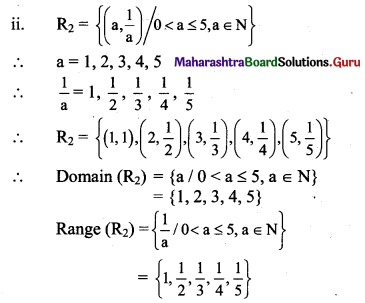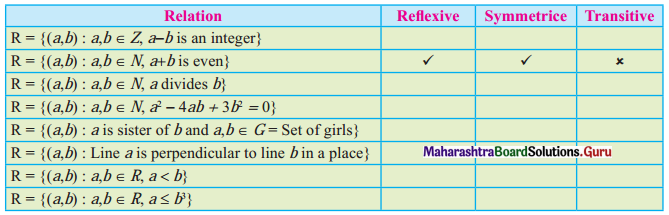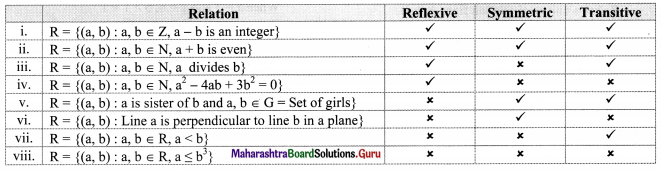Maharashtra Board 11th Maths Solutions Chapter 5 Sets and Relations Ex 5.2

Balbharti Maharashtra State Board 11th Maths Book Solutions Pdf Chapter 5 Sets and Relations Ex 5.2 Questions and Answers.

Maharashtra State Board 11th Maths Solutions Chapter 5 Sets and Relations Ex 5.2

Question 1.
If (x – 1, y + 4) = (1, 2), find the values of x and y.
Solution:
(x – 1, y + 4) = (1, 2)
By the definition of equality of ordered pairs, we have
x – 1 = 1 and y + 4 = 2
∴ x = 2 and y = -2

Question 2.
If $$\left(x+\frac{1}{3}, \frac{y}{3}-1\right)=\left(\frac{1}{2}, \frac{3}{2}\right)$$, find x and y.
Solution:
$$\left(x+\frac{1}{3}, \frac{y}{3}-1\right)=\left(\frac{1}{2}, \frac{3}{2}\right)$$
By the definition of equality of ordered pairs, we have
x + $$\frac{1}{3}$$ = $$\frac{1}{2}$$ and $$\frac{y}{3}$$ – 1 = $$\frac{3}{2}$$
∴ x = $$\frac{1}{2}$$ – $$\frac{1}{3}$$ and $$\frac{y}{3}$$ = $$\frac{3}{2}$$ + 1
∴ x = $$\frac{1}{6}$$ and y = $$\frac{15}{2}$$Question 3.
If A = {a, b, c}, B = {x, y}, find A × B, B × A, A × A, B × B.
Solution:
A = (a, b, c}, B = {x, y}
A × B = {(a, x), (a, y), (b, x), (b, y), (c, x), (c, y)}
B × A = {(x, a), (x, b), (x, c), (y, a), (y, b), (y, c)}
A × A = {(a, a), (a, b), (a, c), (b, a), (b, b), (b, c), (c, a), (c, b), (c, c)}
B × B = {(x, x), (x, y), (y, x), (y, y)}

Question 4.
If P = {1, 2, 3} and Q = {1, 4}, find sets P × Q and Q × P.
Solution:
P = {1, 2, 3}, Q = {1, 4}
∴ P × Q = {(1, 1), (1, 4), (2, 1), (2, 4), (3, 1), (3, 4)}
and Q × P = {(1, 1), (1, 2), (1, 3), (4, 1), (4, 2), (4, 3)}

Question 5.
Let A = {1, 2, 3, 4}, B = {4, 5, 6}, C = {5, 6}. Verify,
(i) A × (B ∩ C) = (A × B) ∩ (A × C)
(ii) A × (B ∪ C) = (A × B) ∪ (A × C)
Solution:
A = {1, 2, 3, 4}, B = {4, 5, 6}, C = {5, 6}
(i) B ∩ C = {5, 6}
A × (B ∩ C) = = {(1, 5), (1, 6), (2, 5), (2, 6), (3, 5), (3, 6), (4, 5), (4, 6)}
A × B = {(1, 4), (1, 5), (1, 6), (2, 4), (2, 5), (2, 6), (3, 4), (3, 5), (3, 6), (4, 4), (4, 5), (4, 6)}
A × C = {(1, 5), (1, 6), (2, 5), (2, 6), (3, 5), (3, 6), (4, 5), (4, 6)}
∴ (A × B) ∩ (A × C) = {(1, 5), (1, 6), (2, 5), (2, 6), (3, 5), (3, 6), (4, 5), (4, 6)}
∴ A × (B ∩ C) = (A × B) ∩ (A × C)

(ii) B ∪ C = {4, 5, 6}
A × (B ∪ C) = {(1, 4), (1, 5), (1, 6), (2, 4), (2, 5), (2, 6), (3,4), (3, 5), (3, 6), (4, 4), (4, 5), (4, 6)}
A × B = {(1, 4), (1, 5), (1, 6), (2, 4), (2, 5), (2, 6), (3, 4), (3, 5), (3, 6), (4, 4), (4, 5), (4, 6)}
A × C = {(1, 5), (1, 6), (2, 5), (2, 6), (3, 5), (3, 6), (4, 5), (4, 6)}
∴ (A × B) ∪ (A × C) = {(1, 4), (1, 5), (1, 6), (2, 4), (2, 5), (2, 6), (3, 4), (3, 5), (3, 6), (4, 4), (4, 5), (4, 6)}
∴ A × (B ∪ C) = (A × B) ∪ (A × C)Question 6.
Express {(x, y) / x2 + y2 = 100, where x, y ∈ W} as a set of ordered pairs.
Solution:
{(x, y) / x2 + y2 = 100, where x, y ∈ W}
We have, x2 + y2 = 100
When x = 0 and y = 10,
x2 + y2 = 02 + 102 = 100
When x = 6 and y = 8,
x2 + y2 = 62 + 82 = 100
When x = 8 and y = 6,
x2 + y2 = 82 + 62 = 100
When x = 10 and y = 0,
x2 + y2 = 102 + 02 = 100
∴ Set of ordered pairs = {(0, 10), (6, 8), (8, 6), (10, 0)}

Question 7.
Let A = {6, 8} and B = {1, 3, 5}. Show that R1 = {(a, b) / a ∈ A, b ∈ B, a – b is an even number} is a null relation, R2 = {(a, b) / a ∈ A, b ∈ B, a + b is an odd number} is a universal relation.
Solution:
A = {6, 8}, B = {1, 3, 5}
R1 = {(a, b)/ a ∈ A, b ∈ B, a – b is an even number}
a ∈ A
∴ a = 6, 8
b ∈ B
∴ b = 1, 3, 5
When a = 6 and b = 1, a – b = 5, which is odd
When a = 6 and b = 3, a – b = 3, which is odd
When a = 6 and b = 5, a – b = 1, which is odd
When a = 8 and b = 1, a – b = 7, which is odd
When a = 8 and b = 3, a – b = 5, which is odd
When a = 8 and b = 5, a – b = 3, which is odd
Thus, no set of values of a and b gives a – b as even.
∴ R1 has a null relation from A to B.
A × B = {(6, 1), (6, 3), (6, 5), (8, 1), (8, 3), (8, 5)}
When a = 6 and b = 1, a + b = 7, which is odd
When a = 6 and b = 3, a + b = 9, which is odd
When a = 6 and b = 5, a + b = 11, which is odd
When a = 8 and b = 1, a + b = 9, which is odd
When a = 8 and b = 3, a + b = 11, which is odd
When a = 8 and b = 5, a + b = 13, which is odd
∴ R2 = {(6, 1), (6, 3), (6, 5), (8, 1), (8, 3), (8, 5)}
Here, R2 = A × B
∴ R2 has a universal relation from A to B.Question 8.
Write the relation in the Roster form. State its domain and range.
(i) R1 = {(a, a2) / a is a prime number less than 15}
(ii) R2 = {(a, $$\frac{1}{a}$$) / 0 < a ≤ 5, a ∈ N}
(iii) R3 = {(x, y / y = 3x, y ∈ {3, 6, 9, 12}, x ∈ {1, 2, 3}}
(iv) R4 = {(x, y) / y > x + 1, x = 1, 2 and y = 2, 4, 6}
(v) R5 = {(x, y) / x + y = 3, x, y ∈ {0, 1, 2, 3}}
(vi) R6 = {(a, b) / a ∈ N, a < 6 and b = 4}
(vii) R7 = {(a, b) / a, b ∈ N, a + b = 6}
(viii) R8 = {(a, b)/ b = a + 2, a ∈ Z, 0 < a < 5}
Solution:
(i) R1 = {(a, a2) / a is a prime number less than 15}
∴ a = 2, 3, 5, 7, 11, 13
∴ a2 = 4, 9, 25, 49, 121, 169
∴ R1 = {(2, 4), (3, 9), (5, 25), (7, 49), (11, 121), (13, 169)}
∴ Domain (R1) = {a/a is a prime number less than 15}
= {2, 3, 5, 7, 11, 13}
Range (R1) = {a2/a is a prime number less than 15}
= {4, 9, 25, 49, 121, 169}(iii) R3 = {(x, y) / y = 3x, x ∈ {1, 2, 3}, y ∈ {3, 6, 9, 12}}
Here y = 3x
When x = 1, y = 3(1) = 3
When x = 2, y = 3(2) = 6
When x = 3, y = 3(3) = 9
∴ R3 = {(1, 3), (2, 6), (3, 9)}
∴ Domain (R3) ={1, 2, 3}
∴ Range (R3) = {3, 6, 9}

(iv) R4 = {(x, y) / y > x + 1, x = 1, 2 and y = 2, 4, 6}
Here, y > x + 1
When x = 1 and y = 2, 2 ≯  1 + 1
When x = 1 and y = 4, 4 > 1 + 1
When x = 1 and y = 6, 6 > 1 + 1
When x = 2 and y = 2, 2 ≯  2 + 1
When x = 2 and y = 4, 4 > 2 + 1
When x = 2 and y = 6, 6 > 2 + 1
∴ R4 = {(1, 4), (1, 6), (2, 4), (2, 6)}
Domain (R4) = {1, 2}
Range (R4) = {4, 6}

(v) R5 = {{x, y) / x + y = 3, x, y ∈ (0, 1, 2, 3)}
Here, x + y = 3
When x = 0, y = 3
When x = 1, y = 2
When x = 2, y = 1
When x = 3, y = 0
∴ R5 = {(0, 3), (1, 2), (2, 1), (3, 0)}
Domain (R5) = {0, 1, 2, 3}
Range (R5) = {3, 2, 1, 0}(vi) R6 = {(a, b)/ a ∈ N, a < 6 and b = 4}
a ∈ N and a < 6
∴ a = 1, 2, 3, 4, 5 and b = 4
R6 = {(1, 4), (2, 4), (3, 4), (4, 4), (5, 4)}
Domain (R6) = {1, 2, 3, 4, 5}
Range (R6) = {4}

(vii) R7 = {(a, b) / a, b ∈ N, a + b = 6}
Here, a + b = 6
When a = 1, b = 5
When a = 2, b = 4
When a = 3, b = 3
When a = 4, b = 2
When a = 5, b = 1
∴ R7 = {(1, 5), (2, 4), (3, 3), (4, 2), (5, 1)}
Domain (R7) = {1, 2, 3, 4, 5}
Range (R7) = {5, 4, 3, 2, 1}

(viii) R8 = {(a, b) / b = a + 2, a ∈ Z, 0 < a < 5}
Here, b = a + 2
When a = 1, b = 3
When a = 2, b = 4
When a = 3, b = 5
When a = 4, b = 6
∴ R8 = {(1, 3), (2, 4), (3, 5), (4, 6)}
Domain (R8) = {1, 2, 3, 4}
Range (R8) = {3, 4, 5, 6}

Question 9.
Identify which of the following relations are reflexive, symmetric, and transitive.Solution:(i) Given, R = {(a, b): a, b ∈ Z, a – b is an integer}
Let a ∈ Z, then a – a ∈ Z
∴ (a, a) ∈ R
∴ R is reflexive.
Let (a, b) ∈ R
∴ a – b ∈ Z
∴ -(a – b) ∈ Z, i.e., b – a ∈ Z
∴ (b, a) ∈ R
∴ R is symmetric.
Let (a, b) and (b, c) ∈ R
∴ a – b ∈ Z and b – c ∈ Z
∴ (a – b) + (b – c) ∈ Z
∴ a – c ∈ Z
∴ (a, c) ∈ R
∴ R is transitive.

(ii) Given, R = {(a, b) : a, b ∈ N, a + b is even}
Let a ∈ N, then a + a = 2a, which is even.
∴ (a, a) ∈ R
∴ R is reflexive.
Let (a, b) ∈ R
∴ a + b is even
∴ b + a is even
∴ (b, a) ∈ R
∴ R is symmetric.
Let (a, b) and (b, c) ∈ R
∴ a + b and b + c is even
Let a + b = 2x and b + c = 2y for x, y ∈ N
∴ (a + b) + (b + c) = 2x + 2y
∴ a + 2b + c = 2(x + y)
∴ a + c = 2(x + y) – 2b = 2(x + y – b)
∴ a + c is even ……..[∵ x, y, b ∈ N, x + y – b ∈ N]
∴ (a, c) ∈ R
∴ R is transitive.(iii) Given, R = {(a, b) : a, b ∈ N, a divides b}
Let a ∈ N, then a divides a.
∴ (a, a) ∈ R
∴ R is reflexive.
Let a = 2 and b = 8, then 2 divides 8
∴ (a, b) ∈ R
But 8 does not divide 2.
∴ (b, a) ∉ R
∴ R is not symmetric.
Let (a, b) and (b, c) ∈ R
∴ a divides b and b divides c.
Let b = ax and c = by for x, y ∈ N.
∴ c = (ax) y = a(xy)
i.e., a divides c.
∴ (a, c) ∈ R
∴ R is transitive.

(iv) Given, R = {(a, b) : a, b ∈ N, a2 – 4ab + 3b2 = 0}
Let a ∈ N, then a2 – 4aa + 3a2 = a2 – 4a2 + 3a2 = 0
∴ (a, a) ∈ R
∴ R is reflexive.
Let a = 3 and b = 1,
then a2 – 4ab + 3b2 = 9 – 12 + 3 = 0
∴ (a, b) ∈ R
Consider, b2 – 4ba + 3a2 = 1 – 12 + 9 = -2 ≠ 0
∴ (b, a) ∉ R
∴ R is not symmetric.
Let a = 3, b = 1 and c = $$\frac{1}{3}$$,
then a2 – 4ab + 3b2 = 9 – 12 + 3 = 0 and
b2 – 4bc + 3c2 = 1 – $$\frac{4}{3}$$ + $$\frac{1}{3}$$ = 1 – 1 = 0
∴ we get (a, b) and (b, c) ∈ R.
Consider, a2 – 4ac + 3c2 = 9 – 4 + $$\frac{1}{3}$$ = $$\frac{16}{3}$$ ≠ 0
∴ (a, c) ∉ R
∴ R is not transitive.

(v) Given, R = {(a, b) : a is sister of b and a, b ∈ G = Set of girls}
Let a ∈ G, then ‘a’ cannot be a sister of herself.
∴ (a, a) ∉ R
∴ R is not reflexive.
Let (a, b) ∈ R
∴ ‘a’ is a sister of ‘b’.
∴ ‘b’ is a sister of ‘a’.
∴ (b, c) ∈ R
∴ R is symmetric.
Let (a, b) and (b, c) ∈ R
∴ ‘a’ is a sister of ‘b’ and ‘b’ is a sister of ‘c’
∴ ‘a’ is a sister of ‘c’.
∴ (a, c) ∈ R
∴ R is transitive.

(vi) Given, R = {(a, b) : Line a is perpendicular to line b in a plane}
Let a be any line in the plane, then a cannot be perpendicular to itself.
∴ (a, a) ∉ R
∴ R is not reflexive.
Let (a, b) ∈ R
∴ a is perpendicular to b.
∴ b is perpendicular to a.
∴ (b, a) ∈ R.
∴ R is symmetric.
Let (a, b) and (b, c) ∈ R.
∴ a is perpendicular to b and b is perpendicular to c.
∴ a is parallel to c.
∴ (a, c) ∉ R
∴ R is not transitive.

(vii) Given, R = {(a, b) : a, b ∈ R, a < b}
Let a ∈ R, then a ≮ a.
∴ (a, a) ∉ R
∴ R is not reflexive.
Let a = 1 and b = 2, then 1 < 2
∴ (a, b) ∈ R
But 2 ≮ 1
∴ (b, a) ∉ R
∴ R is not symmetric.
Let (a, b) and (b, c) ∈ R
∴ a < b and b < c
∴ a < c
∴ (a, c) ∈ R
∴ R is transitive.(viii) Given, R = {(a, b) : a, b ∈ R, a ≤ b3}
Let a = -3, then a3 = -27.
Here, a ≮ a
∴ (a, a) ∉ R
∴ R is not reflexive.
Let a = 2 and b = 9, then b3 = 729
Here, a < b3
∴ (a, b) ∈ R
Consider, a3 = 8
Here, b ≮ a3
∴ (b, a) ∉ R
∴ R is not symmetric.
Let a = 10, b = 3, c = 2,
then b3 = 27 and c3 = 8
Here, a < b3 and b < c3.
∴ (a, b) and (b, c) ∈ R
But a ≮ c3
∴ (a, c) ∉ R.
∴ R is not transitive.